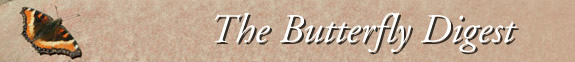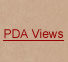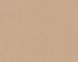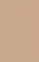Jump to : Today April 1, 2020 March 31, 2020 March 30, 2020 March 29, 2020 March 28, 2020 March 27, 2020 March 26, 2020 March 25, 2020 March 24, 2020 March 23, 2020 March 22, 2020 March 21, 2020 March 20, 2020 March 19, 2020 March 18, 2020 March 17, 2020 March 16, 2020 March 15, 2020 March 14, 2020 March 13, 2020 March 12, 2020 March 11, 2020 March 10, 2020 March 9, 2020 March 8, 2020 March 7, 2020 March 6, 2020 March 5, 2020 March 4, 2020 March 3, 2020     |    View All Lists    |    FAQ
 List: western_monarchs Date: Wed, 25 Mar 2020 07:55:59 -0700 From: Home [western_monarchs] Subject: =?utf-8?Q?Re:_[western=5Fmonarchs]_What=E2=80=99s_Happening_to_the?= =?utf-8?Q?_=28Western=29_Monarch_Butterfly_Population=3F_=28New_York_Tim?= =?utf-8?Q?es,_March_20/20=29?= --Apple-Mail-24BA8AF1-BAF1-4A0E-9E16-3886EB33B68DContent-Type: text/plain; charset=utf-8Content-Transfer-Encoding: quoted-printableThis kind of discussion is this group at its best! Thank you! Mia=20Sent from my iPhone> On Mar 24, 2020, at 9:43 PM, Francis Xavier Villablanca footlooseandfranc= [western_monarchs] wrot=e:>=20> =EF=BB=BF=20>=20> Hi all,>=20> Paul, your comment is appreciated. In the same volume that Nagano et al. =published their paper arguing for non-random spring remigration dispersal, =Wenner (and Harris) argued that monarchs do not migrate. Clearly, at that t=ime, there was disagreement. Even if Lincoln was convincingly persuaded, wi=thout seeing Wenner's arguments it is hard to agree or disagree. Though Lin=coln cites Wenner 1994, this was only personal correspondence. There is no =evidence of what the "reanalysis" was and therefore it is impossible to kno=w if it was or was not valid. This is important because in general acceptin=g a hypothesis of randomness (as Wenner would have done) is quite difficult=.. For example, one could fail to see a pattern simply due to a lack of suff=icient data, or due to using a statistical method that lacks power to resol=ve that pattern. In contrast rejecting randomness is quite convincing. That= is a fundamental premise of statistical testing. So without Wenner's work =to evaluate the best we have is Nagano et al's conclusion.>=20> Just wanted to set the record straight.>=20> Be well, Francis Villablanca> San Luis Obispo=20>=20>> On Tue, Mar 24, 2020 at 6:04 PM Paul Cherubini [wester=n_monarchs] wrote:>> Francis:>>=20>> =E2=80=9CIn regards to "where do they go." Some data have been=20>> interpreted as showing that movement is random. I would>> caution against accepting that interpretation if there has=20>> been no statistical analysis. For example, Nagano at al.=20>> 1993 show that recaptured tags from overwintering monarchs=20>> leaving the coast have [a] pattern [that] is non-random.=20>> They conclude that on average the monarch butterflies=20>> they tagged moved in a Northwesterly (326*) and in an=20>> Easterly (99*) directions. Figure 3 shows that movement=20>> is not just in those two directions. There is variation. But=20>> the variation is not random.=E2=80=9D>>=20>> In 1994 UC Santa Barbara professor Adrian Wenner reanalyzed=20>> Nagano et al.=E2=80=99s 1993 spring migration dispersal from the coast>> tag recapture data and, to quote Dr. Lincoln Brower:>> =E2=80=9Cconvincingly argued that the purported bidirectional=20>> distribution can not be statistically differentiated=20>> from random headings.=E2=80=9D>>=20>> https://imagizer.imageshack.com/img924/724/Nm5dJT.jpg>>=20>> Paul Cherubini>> El Dorado, Calif.>=20>=20>=20--Apple-Mail-24BA8AF1-BAF1-4A0E-9E16-3886EB33B68DContent-Type: text/html; charset=UTF-8Content-Transfer-Encoding: quoted-printable

This kind of discussion is this group at its best!  Thank you!  M=ia

Sent from my iPhone
=
On Mar 24, 2020, at 9:43 PM, Francis Xavier V=illablanca [western_monarchs] <western=<_monarchs...>> wrote:

=EF=BB=BF=20=20=20=20=20=20=20=20
Hi all,

Paul, your comment is appreciat=ed. In the same volume that Nagano et al. published their paper arguin=g for non-random spring remigration dispersal, Wenner (and Harris) argued t=hat monarchs do not migrate. Clearly, at that time, there was disagreement.= Even if Lincoln was convincingly persuaded, without seeing Wenner's argume=nts it is hard to agree or disagree. Though Lincoln cites Wenner 1994, this= was only personal correspondence. There is no evidence of what the "reanal=ysis" was and therefore it is impossible to know if it was or was not valid=.. This is important because in general accepting a hypothesis of randomness= (as Wenner would have done) is quite difficult. For example, one could fai=l to see a pattern simply due to a lack of sufficient data, or due to using= a statistical method that lacks power to resolve that pattern. In contrast= rejecting randomness is quite convincing. That is a fundamental premise of= statistical testing. So without Wenner's work to evaluate the best we =;have is Nagano et al's conclusion.

Just wanted to= set the record straight.

Be well, Francis Villabl=anca
San Luis Obispo

On Tue, Mar 24, 2020 at 6:04 PM P=aul Cherubini "> [w=estern_monarchs] <">we=> wrote:
=20

=20=20=20=20=20=20=20=20=20=20=20=20

Francis:

==E2=80=9CIn regards to "where do they go." Some data have been =
interpreted =as showing that movement is random. I would
caution against accepting that interp=retation if there has
been no statistical analysis. For examp=le, Nagano at al.
1993 show that recaptured tags from overwintering monarchs&nbs=p;
leaving&=nbsp;the coast have [a] pattern [that] is non-random.
They conclude that on average the monarch butterflies
they tagged moved in a Northweste=rly (326*) and in an
Easterly (99*) directions. Figure 3 shows that movemen=t
is =not just in those two directions. There is variation. But&=nbsp;
the v=ariation is not random.=E2=80=9D

In 1994 UC Santa Barbara professor Adrian W=enner reanalyzed
Nagano et al.=E2=80=99s 1993 spring migration dispersal from th=e coast
tag= recapture data and, to quote Dr. Lincoln Brower:
=E2=80=9Cconvincingly argued that the= purported bidirectional
distribution can not be statistically differentiated

Paul Cherubini
El Dora=do, Calif.

=20=20=20=20=20=20=20=20=20
=20=20

__._,_.___
=20=20=20=20=20=20=20=20=20=20=20=20

Posted by: Home <>
=
=20=20=20=20
=20=20=20=20=20=20=20=20=20 =20=20=20
__,_._,___
--Apple-Mail-24BA8AF1-BAF1-4A0E-9E16-3886EB33B68D--